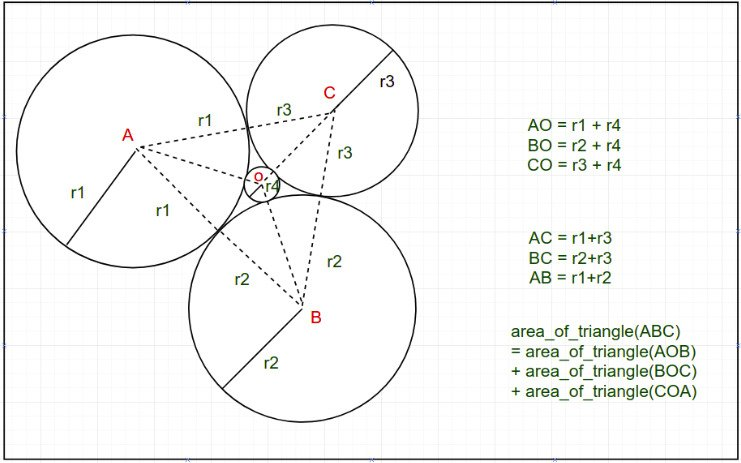# Descartes’ Circle Theorem with implementation

• Last Updated : 23 Jul, 2021

In geometry, Descartes’ theorem states that for every four mutually tangent circles, the radii of the circles satisfy a certain quadratic equation. One can construct a fourth circle tangent to three given, mutually tangent circles.
The Descartes’ Circle Theorem helps us to find the radius of a circle when there are 4 circles with positive integer radius r1, r2, r3 and r4 as shown in the figure below. It finds the radius r4 of the circle formed by three circles of radius r1, r2, r3 as shown in the image below.
(Note that the circles in the picture below are tangent to each other.)Attention reader! Don’t stop learning now. Get hold of all the important DSA concepts with the DSA Self Paced Course at a student-friendly price and become industry ready.  To complete your preparation from learning a language to DS Algo and many more,  please refer Complete Interview Preparation Course.

In case you wish to attend live classes with experts, please refer DSA Live Classes for Working Professionals and Competitive Programming Live for Students.

Examples:

Input: r1 = 1, r2 = 1, r3 = 1
Output: 0.154701
Input: r1 =23, r2 = 46, r3 = 69
Output: 6.000000

The theorem says that the reciprocals of radii, or “curvatures”, of these circlessatisfies the following relation:One of the circles can be enclosing among all the remaining three, as in the figure, in which case the corresponding curvaturehere is considered negative and the above relation still holds.
Ifare known, one can solve for k4,On solving the above equation we get the radius of the fourth circle. The formula for finding the fourth circle’s radius is:Thus if r1, r2, and r3 are known, r4 can easily be calculated using the above formula.
Below is the implementation of the above approach:

## CPP

 // C++ implementation of the// above formulae#include using namespace std; // Function to find the fourth circle's// when three radius are givendouble findRadius(double r1, double r2, double r3){    double r4 = (r1 * r2 * r3)                / (r1 * r2 + r2 * r3                   + r1 * r3 + 2.0 * sqrt(r1 * r2 * r3 * (r1 + r2 + r3)));     return r4;} // Driver codeint main(){    // Radius of three circles    double r1 = 1;    double r2 = 1;    double r3 = 1;     // Calculation of r4 using formula given above    double r4 = findRadius(r1, r2, r3);     cout << "The radius of fourth circle: " << r4;    return 0;}

## Java

 /*package whatever //do not write package name here */// Java implementation of the// above formulaeimport java.io.*; class GFG{           // Function to find the fourth circle's        // when three radius are given        static double findRadius(double r1, double r2, double r3)        {        double r4 = (r1 * r2 * r3)                / (r1 * r2 + r2 * r3                   + r1 * r3 + 2.0 * Math.sqrt(r1 * r2 * r3 * (r1 + r2 + r3)));         return r4;        }         // Driver code    public static void main (String[] args)    {               // Radius of three circles    double r1 = 1;    double r2 = 1;    double r3 = 1;     // Calculation of r4 using formula given above    double r4 = findRadius(r1, r2, r3);        System.out.println("The radius of fourth circle: " + r4);    }} // This code is contributed by CoderSaty.

## Python3

 # Python 3 implementation of the# above formulae from math import sqrt # Function to find the fourth circle's# when three radius are givendef findRadius(r1, r2, r3):    r4 = (r1 * r2 * r3) / (r1 * r2 + r2 * r3 + r1 * r3 + 2.0 * sqrt(r1 * r2 * r3 * (r1 + r2 + r3)))     return r4 # Driver codeif __name__ == '__main__':       # Radius of three circles    r1 = 1    r2 = 1    r3 = 1     # Calculation of r4 using formula given above    r4 = findRadius(r1, r2, r3)     print("The radius of fourth circle:",r4)         # This code is contributed by SURENDRA_GANGWAR.

## C#

 // C# implementation of the// above formulaeusing System; class GFG{   // Function to find the fourth circle's  // when three radius are given  static double findRadius(double r1, double r2, double r3)  {    double r4 = (r1 * r2 * r3)      / (r1 * r2 + r2 * r3         + r1 * r3 + 2.0 * Math.Sqrt(r1 * r2 * r3 * (r1 + r2 + r3)));     return r4;  }   // Driver code  public static void Main (String[] args)  {     // Radius of three circles    double r1 = 1;    double r2 = 1;    double r3 = 1;     // Calculation of r4 using formula given above    double r4 = Math.Round(findRadius(r1, r2, r3),6);     Console.Write("The radius of fourth circle: " + r4);  }} // This code is contributed by shivanisinghss2110

## Javascript

 
Output:
The radius of fourth circle: 0.154701

My Personal Notes arrow_drop_up